# Introduction to gghighlight

## Motivation

Suppose we have data that has so many series that it is hard to identify them by their colours as the differences are so subtle.

``````set.seed(2)
d <- purrr::map_dfr(
letters,
~ data.frame(
idx = 1:400,
value = cumsum(runif(400, -1, 1)),
type = .,
flag = sample(c(TRUE, FALSE), size = 400, replace = TRUE),
stringsAsFactors = FALSE
)
)``````
``````library(ggplot2)

ggplot(d) +
geom_line(aes(idx, value, colour = type))``````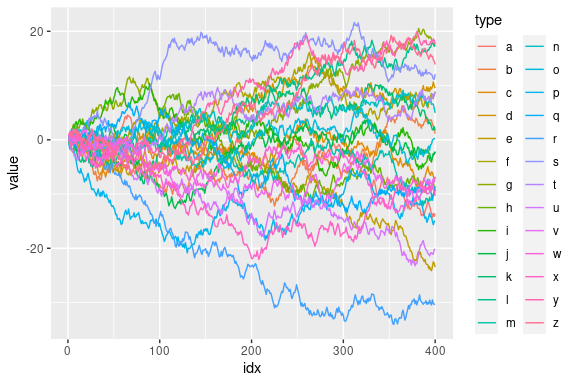To filter the data to a reasonable number of lines, we can use dplyr’s `filter()`.

``````library(dplyr, warn.conflicts = FALSE)

d_filtered <- d %>%
group_by(type) %>%
filter(max(value) > 20) %>%
ungroup()

ggplot(d_filtered) +
geom_line(aes(idx, value, colour = type))``````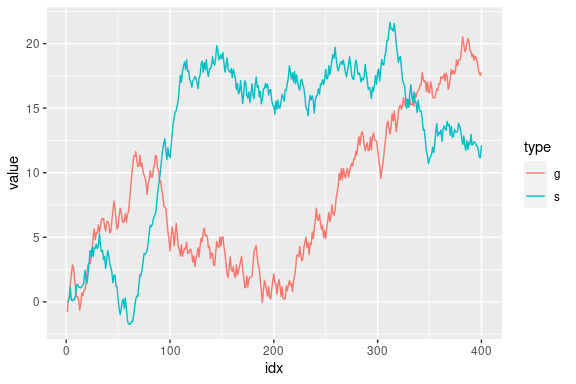But, it seems not so handy. For example, what if we want to change the threshold in predicate (`max(value) > 20`) and highlight other series as well? It’s a bit tiresome to type all the code above again every time we replace `20` with some other value.

Besides, considering one of the main purposes of visualization is to get the overview of a data, it may not be good to simply filter out the unmatched data because the plot will lose its context.

Here comes gghighlight package, `dplyr::filter()` equivalent for ggplot2.

(If you are interested in more details behind the idea of highlighting, please read this post: Anatomy of gghighlight.)

## gghighlight()

The main function of the gghighlight package is `gghighlight()`. For example, by using this function, we can highlight the lines whose max values are larger than 20 as seen below:

``````library(gghighlight)

ggplot(d) +
geom_line(aes(idx, value, colour = type)) +
gghighlight(max(value) > 20)
#> label_key: type``````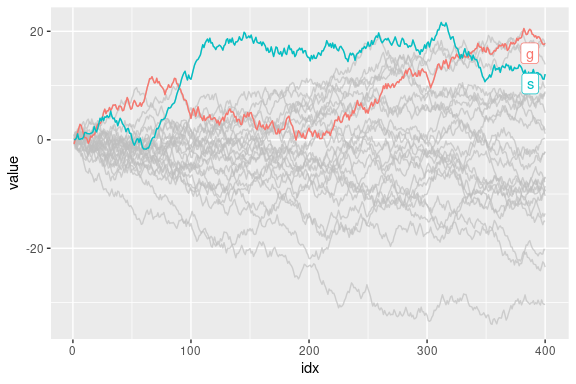You can specify as many predicates as you like. For example, the following code highlights the data that satisfies both `max(value) > 15` and `mean(flag) > 0.55`.

``````ggplot(d) +
geom_line(aes(idx, value, colour = type)) +
gghighlight(max(value) > 15, mean(flag) > 0.55)
#> label_key: type``````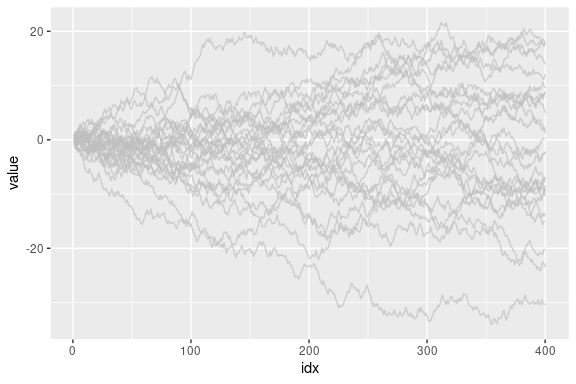## Customization

As adding `gghighlight()` results in a ggplot object, it is fully customizable just as we usually do with ggplot2 like custom themes.

``````ggplot(d) +
geom_line(aes(idx, value, colour = type)) +
gghighlight(max(value) > 19) +
theme_minimal()
#> label_key: type``````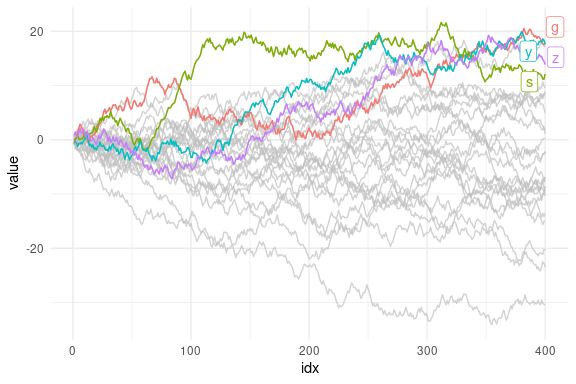The plot also can be facetted:

``````ggplot(d) +
geom_line(aes(idx, value, colour = type)) +
gghighlight(max(value) > 19) +
theme_minimal() +
facet_wrap(~ type)
#> label_key: type``````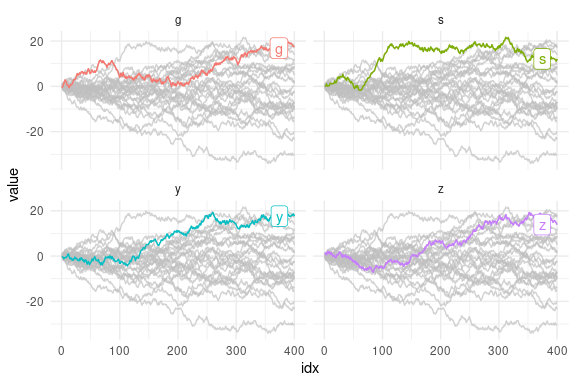There are also some options to control the way of highlighting. See “Options” section below.

## Geoms

`gghighlight()` can highlight almost every geom. Here are some examples.

### Bar

`gghighlight()` can highlight bars.

``````p <- ggplot(iris, aes(Sepal.Length, fill = Species)) +
geom_histogram() +
gghighlight()
#> label_key: Species

p
#> `stat_bin()` using `bins = 30`. Pick better value with `binwidth`.
#> `stat_bin()` using `bins = 30`. Pick better value with `binwidth`.``````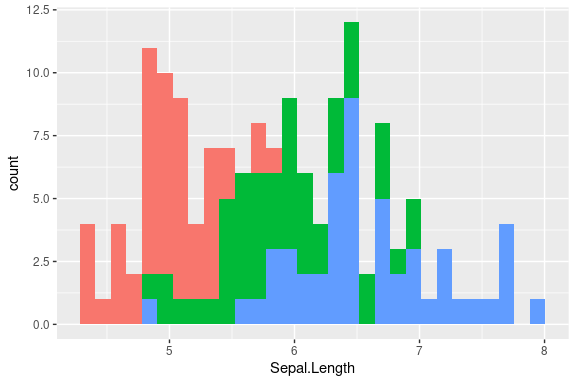Are you wondering if this is really highlighted? Yes, it is. But, the unhighlighted bars are all overwritten by the highlighted bars. This seems not so useful, until you see the facetted version:

``````p + facet_wrap(~ Species)
#> `stat_bin()` using `bins = 30`. Pick better value with `binwidth`.
#> `stat_bin()` using `bins = 30`. Pick better value with `binwidth`.``````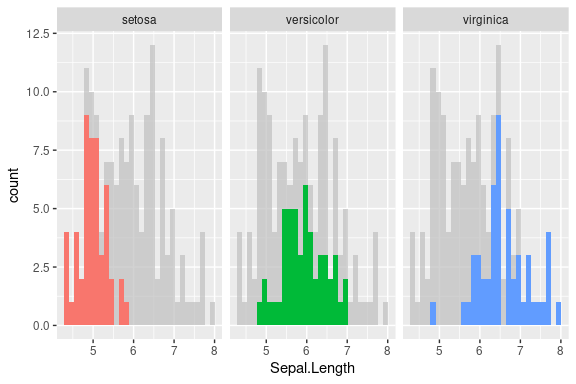### Point

As is explained in Anatomy of gghighlight, lines and points typically have different semantics (group-wise or not). But, in most cases, you don’t need to be careful about the difference with `gghighlight()` because it automatically picks the right method of calculation.

``````set.seed(10)
d2 <- dplyr::slice_sample(d, n = 20)

ggplot(d2, aes(idx, value)) +
geom_point() +
gghighlight(value > 0, label_key = type)``````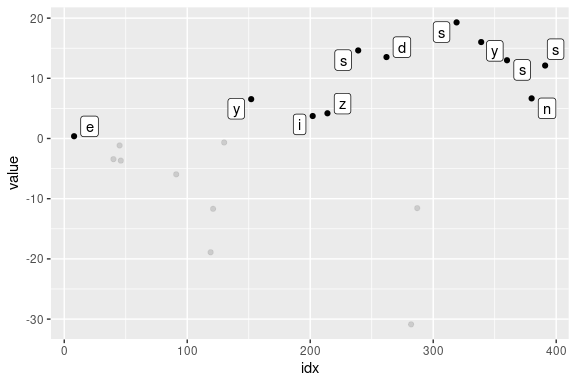More precisely, `gghighlight()` takes the following strategy:

1. Calculate the group IDs from mapping.
1. If `group` exists, use it.
2. Otherwise, assign the group IDs based on the combination of the values of discrete variables.
2. If the group IDs exists, evaluate the predicates in a grouped manner.
3. If the group IDs doesn’t exist or the grouped calculation fails, evaluate the predicates in an ungrouped manner.

Note that, in this case, `label_key = type` is needed to show labels because `gghighlight()` chooses a discrete variable from the mapping, but `aes(idx, value)` consists of continuous variables only.

## Non-logical predicate

To construct a predicate expression like below, we need to determine a threshold (in this example, `20`). But it is difficult to choose a nice one before we draw plots.

``max(value) > 20``

So, `gghighlight()` allows predicates that return non-logical (e.g. numeric and character) results. The values are used for sorting data and the top `max_highlight` of rows/groups are highlighted:

``````ggplot(d, aes(idx, value, colour = type)) +
geom_line() +
gghighlight(max(value), max_highlight = 5L)
#> label_key: type``````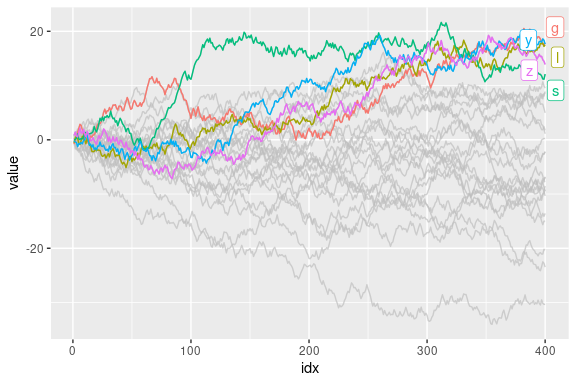## Labels

`gghighlight()` adds direct labels for some geoms. Currently, the following geoms are supported:

• `point`: add labels at each highlighted point.
• `line`: add labels at the right end of each highlighted line.
• `bar`: (do not add labels)

If you don’t want them to be labelled automatically, you can specify `use_direct_label = FALSE`

``````ggplot(d) +
geom_line(aes(idx, value, colour = type)) +
gghighlight(max(value) > 20, use_direct_label = FALSE)``````